# Right Triangle Formulas, Pythagoras, Cathetus, Hypotenuse, Altitude, Projection, Inradius, Circumradius, Exradius, Semiperimeter, Area, Special Right Triangles, Poncelet, 3-D, Coordinate

 PYTHAGOREAN THEOREM, GEOMETRIC MEAN, PRODUCT OF THE CATHETUS, ALTITUDE, PROJECTION: Proofs that use similarity.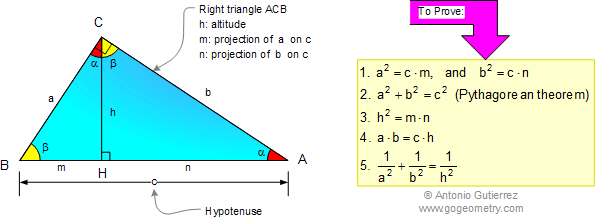SPECIAL RIGHT TRIANGLES: Isosceles 45-45, 30-60, 37-53 (3-4-5)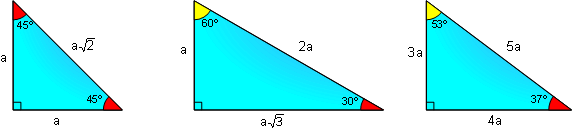CENTERS, INRADIUS, CIRCUMRADIUS, INCENTER, CIRCUMCENTER, ORTHOCENTER, CENTROID, PONCELET'S THEOREM, SAGITTA. Sagitta: The distance between the midpoint of an arc and the midpoint of its chord.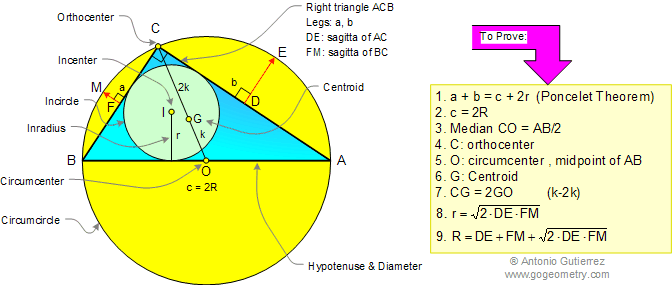RIGHT TRIANGLE: INRADIUS, EXRADII, SEMIPERIMETER (s), CATHETUS AND HYPOTENUSE, AREA.RIGHT TRIANGLE: ORTHOGONAL PROJECTIONS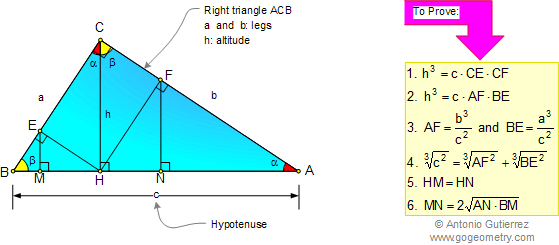RIGHT TRIANGLE: ALTITUDE, INRADII, INCENTER, AREAS.PYTHAGOREAN THEOREM: DIAGONAL OF A BOXDISTANCE IN CARTESIAN COORDINATE: The Pythagorean Theorem provides an easy way to compute the straight line distance between any two points whose Cartesian coordinates are known.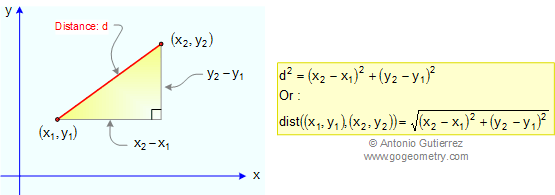PYTHAGOREAN THEOREM IN THREE DIMENSIONS 3-D, De Gua's Theorem: In any tetrahedron with a cubic vertex O-ABC the square of the area of the face opposite the cubic vertex O-ABC is equal to the sum of the squares of the areas of the other three faces AOB, AOC, BOC.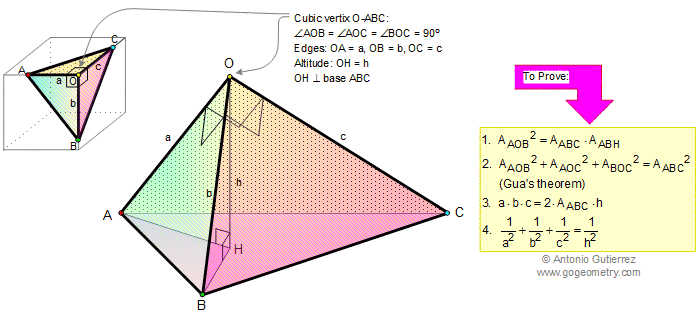THE PYTHAGOREAN CURIOSITY: Triangles and squares, fifteen conclusions. THE GENERAL EXTENSION TO PYTHAGORAS' THEOREM: If any 3 similar shapes are drawn on the sides of a right triangle, then the area of the shape on the hypotenuse equals the sum of the areas on the other two sides.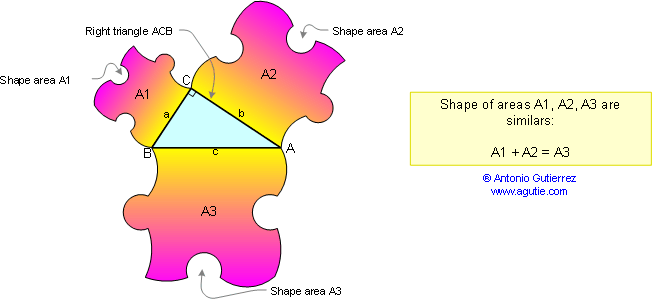Home | Geometry | Pythagoras | Email | By Antonio Gutierrez